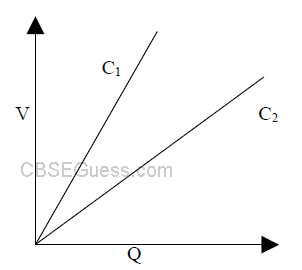# Electrostatics

View Solutions

1. Where the energy of capacitor does resides?

2. Do electrons tend to go to region of low or high potential?

3. What is the net charge on the charged capacitor?

4. A Gaussian surface encloses an electric dipole within it. What is the total flux across sphere?

5. Find the dimension of 1/2εoE2.

6. In a certain l m3 of space, electric potential is found to be V Volt throughout. What is the electric field in this Region?

7. If Coulomb law involves 1/r3 instead of 1/r2 dependence, would Gauss law be still true?

8. An electrostatic field line can’t be discontinuous, why?

9. The given graph shows that the variation of charge versus potential difference V for the two capacitors C1 & C2. The two capacitors have same plate separation but the plate area of C2 is doubled than that of C1.Which of the line in the graph corresponds to C1 &C2 and why?10. Three charges, each equal to +2C are placed at the corners of an equilateral triangle. If the force between any two charges be F, then what will be the net force on either Charge?

11. A point charge q is placed at O as shown in the figure. Is VP-VQ +ve or –ve when (i) q>0, (ii) q<0? Justify your answer.

12. An electric dipole of dipole moment 20 X 10-6 C.m is enclosed by a closed surface. What is the net flux coming out of the surface?

13. Why does the electric field inside a dielectric decrease when it is placed in an external electric field?

14. Write the magnitude and direction of electric field intensity due to an electric dipole of length 2a at the mid point of the line joining the two charges

15. A spherical portion has been removed from a solid sphere having a charge distributed uniformly in its volume as shown in fig. What is the electric field inside the emptied sphere?

16. A charged particle is free to move in an electric field. Will it always move along an electric line of force?

17. If V (=q/4πεor) is the potential at a distance r due to a point charge q, then determine the electric field due to a point charge q, at a distance r.

18. Can electric potential at any point in space be zero while intensity of electric field at that point is not zero?

19. Devise an arrangement of three point charges separated by finite distances that has zero electric potential energy.

20. Each of the uncharged capacitor in the fig. Has a capacitance of 25μF. What charge shall flow through the meter M when the switch S is Closed?

21. Charge of 2C is placed at the centre of a cube of volume 8 cm3. What is the electric flux passing through one face?

22. A charged particle q is shot towards another charged particle Q which is fixed, with a speed v. It approaches Q up to a closest distance r and then returns. If q were given a speed 2v, then find the closest distance of approach.

23. Two capacitors of capacitance 6mF and 12mF are connected in series with the battery. The voltage across the 6mF capacitor is 2 volt .Compute the total battery voltage.

24. A parallel plate capacitor with air between the plates has a capacitance of 8 pF . The separation between the plates is now reduced by half and the space between them is filled with a medium of dielectric constant 5. Calculate the value of capacitance of parallel plate capacitor in second case.

25. Five identical capacitors, each of capacitance C are connected between points X and Y as shown in the figure. If the equivalent capacitance of the combination between X and Y is 5mF. Calculate the capacitance of each capacitor.

26. An uncharged capacitor is connected to a battery. Show that half of the energy supplied by the battery is lost as heat while charging the capacitor.

27. What is the angle between the electric dipole moment and electric field strength due to it on the equilateral line?

28. Find the equivalent capacitance between A & B, if capacitance of each capacitor is C.

29. Eight identically charged drops are joined to form bigger drop. By what factor the charge and potential change?

30. A uniform electric field of 2 kNC-1 is in the x-direction. A point charge of 3 μC initially at rest at the origin is released. What is the kinetic energy of this charge at x = 4m?

31. Two identical metal plates are given the charges Q1 and Q2 (Q2 < Q1) respectively. If they are now brought close together to form a parallel plate capacitor with capacitance C then what is the potential difference between them?

32. Three charges Q, +q and +q are placed at the vertices of a right angle isosceles triangle as shown. Find the magnitude of Q for which net electrostatic energy of the configuration is zero.

33. An infinite number of charges each having charge ‘q’ along x-axis at x=1 ,x=2, x=4 ,x=8 an so on. Find the electric field at x=0 due to these charges.

34. A charge Q is distributed over the two concentric hollow spheres of radii ‘r’ and ‘R’ (R>r) such that the surface densities are equal. Find the potential at the common centre.

35. An electric dipole is held in an uniform electric field. Using suitable diagram, show that it doesn’t undergo any translatory motion, and (ii)Derive an expression for torque acting on it and specify its direction.

36. The field potential inside a charged ball depends only on the distance from its centre as V=ar2+b,where a and b are constants. Find the space charge distribution r(r) inside the ball.

CBSE Physics (Chapter Wise With Hint / Solution) Class XII (By Mr. Sreekumaran Nair)